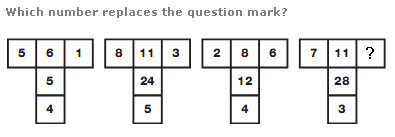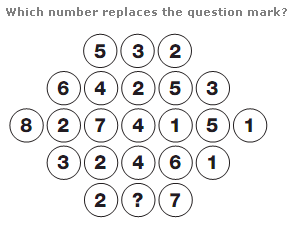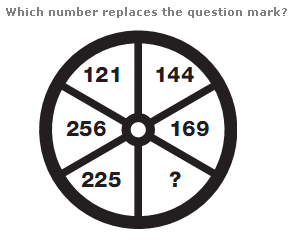# Puzzles - Number puzzles

### Exercise :: Number puzzlesAnswer : 4 Explanation : In each shape, use the left and right hand numbers as a source. The upper central number equals the sum of the left and right hand numbers, the middle central number equals the product of the left and right hand numbers, and the lower central number equals the difference between the left and right hand numbers.Answer : 5 Explanation : Working in rows, in each row the central number is equal to the difference between the sum of the even numbers in the row and the sum of the odd numbers in the row.Answer : 196 Explanation : Starting with the top left segment, and moving clockwise around the circle, numbers follow the sequence of square numbers, from 121 to 256.